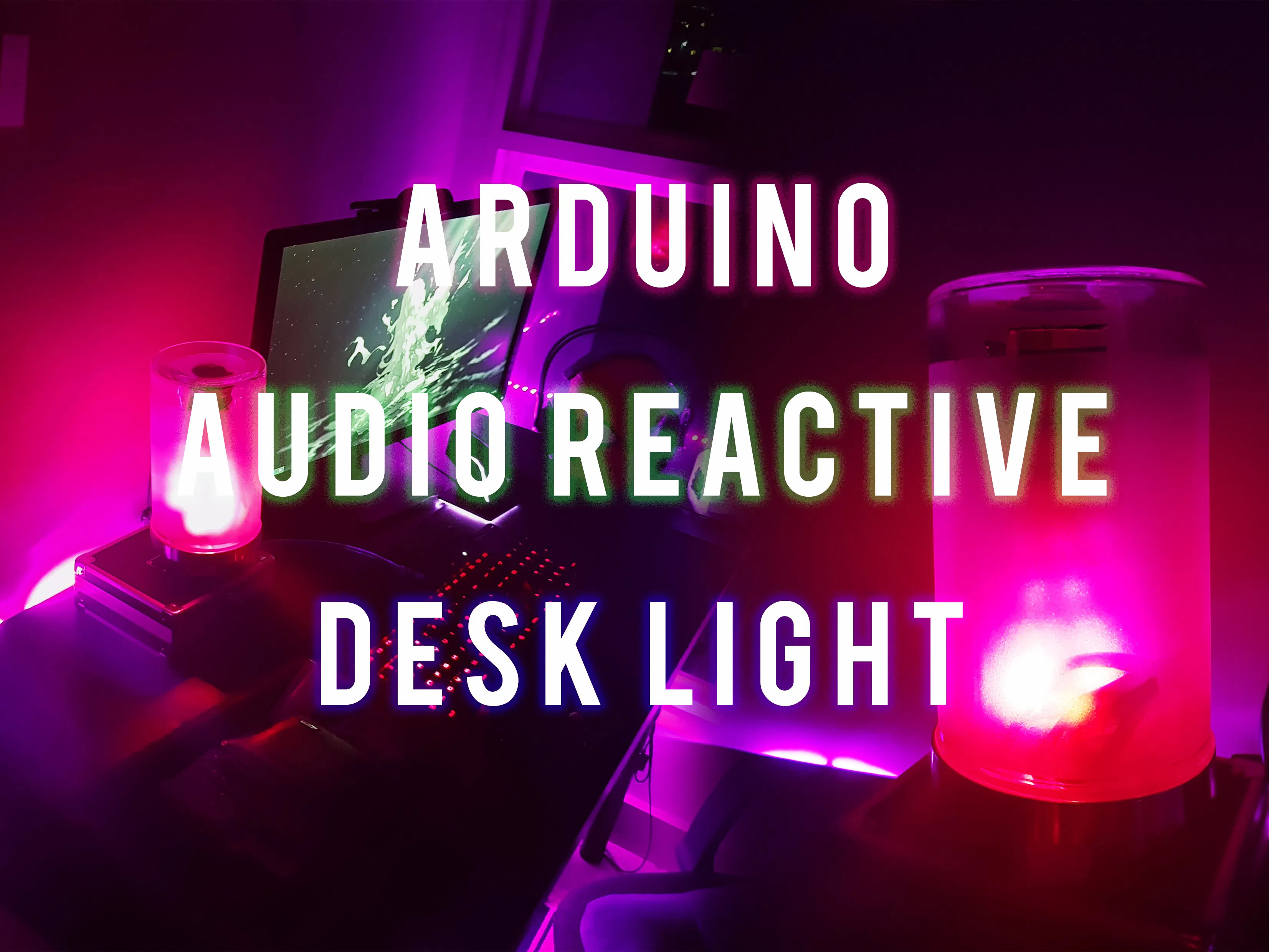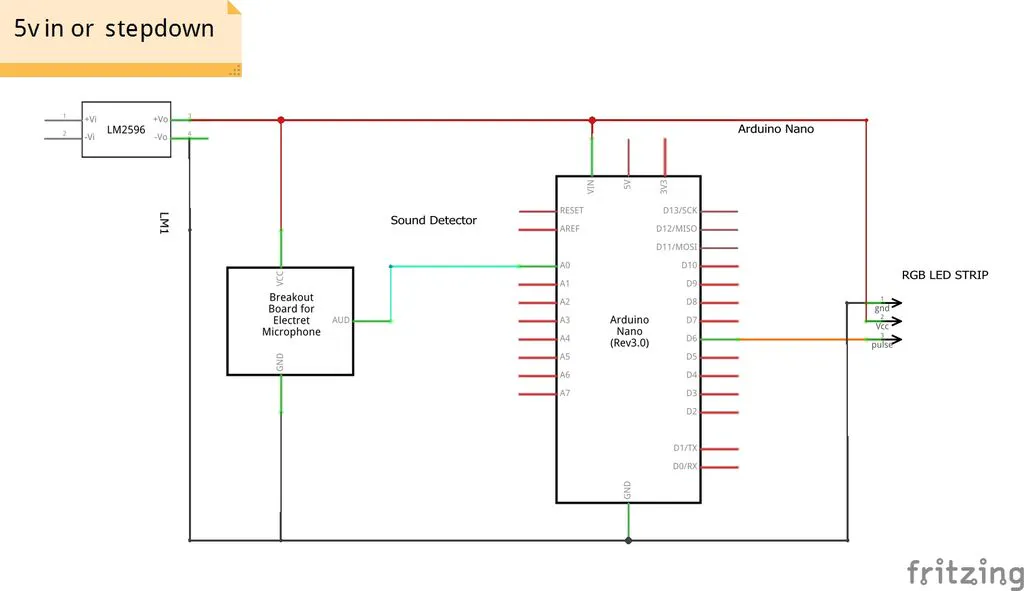# Arduino Audio Reactive Desk Light

It is the amazing RGB LED desk light. It will be reacting on the surrounding sound and music and will be playing like a visualizer.

IntermediateFull instructions provided23,491## Things used in this project

### Hardware componentsArduino Nano R3
×1
 sound module
×1×1Jumper wires (generic)
×1
×1
 Ikea DROPPAR Jar
×1
 A small length of PVC pipe
×1

### Software apps and online servicesArduino IDE

### Hand tools and fabrication machinesSoldering iron (generic)

## Schematics

### Schematic Diagram## Code

### Coding For the Arduino

Arduino
This code will enable the LED to react to the sound that being detected by the sound module.
```#include <FastLED.h>

/** BASIC CONFIGURATION  **/

//The amount of LEDs in the setup
#define NUM_LEDS 60
//The pin that controls the LEDs
#define LED_PIN 6
//The pin that we read sensor values form

//Confirmed microphone low value, and max value
#define MIC_LOW 0.0
#define MIC_HIGH 200.0
/** Other macros */
//How many previous sensor values effects the operating average?
#define AVGLEN 5
//How many previous sensor values decides if we are on a peak/HIGH (e.g. in a song)
#define LONG_SECTOR 20

//Mneumonics
#define HIGH 3
#define NORMAL 2

//How long do we keep the "current average" sound, before restarting the measuring
#define MSECS 30 * 1000
#define CYCLES MSECS / DELAY

/*Sometimes readings are wrong or strange. How much is a reading allowed
to deviate from the average to not be discarded? **/
#define DEV_THRESH 0.8

//Arduino loop delay
#define DELAY 1

float fscale( float originalMin, float originalMax, float newBegin, float newEnd, float inputValue, float curve);
void insert(int val, int *avgs, int len);
int compute_average(int *avgs, int len);
void visualize_music();

//How many LEDs to we display
int curshow = NUM_LEDS;

/*Not really used yet. Thought to be able to switch between sound reactive
mode, and general gradient pulsing/static color*/
int mode = 0;

//Showing different colors based on the mode.
int songmode = NORMAL;

//Average sound measurement the last CYCLES
unsigned long song_avg;

//The amount of iterations since the song_avg was reset
int iter = 0;

//The speed the LEDs fade to black if not relit

//Led array
CRGB leds[NUM_LEDS];

/*Short sound avg used to "normalize" the input values.
We use the short average instead of using the sensor input directly */
int avgs[AVGLEN] = {-1};

//Longer sound avg
int long_avg[LONG_SECTOR] = {-1};

//Keeping track how often, and how long times we hit a certain mode
struct time_keeping {
unsigned long times_start;
short times;
};

//How much to increment or decrement each color every cycle
struct color {
int r;
int g;
int b;
};

struct time_keeping high;
struct color Color;

void setup() {
Serial.begin(9600);
//Set all lights to make sure all are working as expected
for (int i = 0; i < NUM_LEDS; i++)
leds[i] = CRGB(0, 0, 255);
FastLED.show();
delay(1000);

//bootstrap average with some low values
for (int i = 0; i < AVGLEN; i++) {
insert(250, avgs, AVGLEN);
}

//Initial values
high.times = 0;
high.times_start = millis();
Color.r = 0;
Color.g = 0;
Color.b = 1;
}

/*With this we can change the mode if we want to implement a general
lamp feature, with for instance general pulsing. Maybe if the
sound is low for a while? */
void loop() {
switch(mode) {
case 0:
visualize_music();
break;
default:
break;
}
delay(DELAY);       // delay in between reads for stability
}

/**Funtion to check if the lamp should either enter a HIGH mode,
or revert to NORMAL if already in HIGH. If the sensors report values
that are higher than 1.1 times the average values, and this has happened
more than 30 times the last few milliseconds, it will enter HIGH mode.
TODO: Not very well written, remove hardcoded values, and make it more
reusable and configurable.  */
void check_high(int avg) {
if (avg > (song_avg/iter * 1.1))  {
if (high.times != 0) {
if (millis() - high.times_start > 200.0) {
high.times = 0;
songmode = NORMAL;
} else {
high.times_start = millis();
high.times++;
}
} else {
high.times++;
high.times_start = millis();

}
}
if (high.times > 30 && millis() - high.times_start < 50.0)
songmode = HIGH;
else if (millis() - high.times_start > 200) {
high.times = 0;
songmode = NORMAL;
}
}

//Main function for visualizing the sounds in the lamp
void visualize_music() {
int sensor_value, mapped, avg, longavg;

//Actual sensor value

//If 0, discard immediately. Probably not right and save CPU.
if (sensor_value == 0)
return;

mapped = (float)fscale(MIC_LOW, MIC_HIGH, MIC_LOW, (float)MIC_HIGH, (float)sensor_value, 2.0);
avg = compute_average(avgs, AVGLEN);

if (((avg - mapped) > avg*DEV_THRESH)) //|| ((avg - mapped) < -avg*DEV_THRESH))
return;

//Insert new avg. values
insert(mapped, avgs, AVGLEN);
insert(avg, long_avg, LONG_SECTOR);

//Compute the "song average" sensor value
song_avg += avg;
iter++;
if (iter > CYCLES) {
song_avg = song_avg / iter;
iter = 1;
}

longavg = compute_average(long_avg, LONG_SECTOR);

//Check if we enter HIGH mode
check_high(longavg);

if (songmode == HIGH) {
Color.r = 5;
Color.g = 3;
Color.b = -1;
}
else if (songmode == NORMAL) {
Color.r = -1;
Color.b = 2;
Color.g = 1;
}

//Decides how many of the LEDs will be lit
curshow = fscale(MIC_LOW, MIC_HIGH, 0.0, (float)NUM_LEDS, (float)avg, -1);

/*Set the different leds. Control for too high and too low values.
Fun thing to try: Dont account for overflow in one direction,
some interesting light effects appear! */
for (int i = 0; i < NUM_LEDS; i++)
//The leds we want to show
if (i < curshow) {
if (leds[i].r + Color.r > 255)
leds[i].r = 255;
else if (leds[i].r + Color.r < 0)
leds[i].r = 0;
else
leds[i].r = leds[i].r + Color.r;

if (leds[i].g + Color.g > 255)
leds[i].g = 255;
else if (leds[i].g + Color.g < 0)
leds[i].g = 0;
else
leds[i].g = leds[i].g + Color.g;

if (leds[i].b + Color.b > 255)
leds[i].b = 255;
else if (leds[i].b + Color.b < 0)
leds[i].b = 0;
else
leds[i].b = leds[i].b + Color.b;

//All the other LEDs begin their fading journey to eventual total darkness
} else {
}
FastLED.show();
}
//Compute average of a int array, given the starting pointer and the length
int compute_average(int *avgs, int len) {
int sum = 0;
for (int i = 0; i < len; i++)
sum += avgs[i];

return (int)(sum / len);

}

//Insert a value into an array, and shift it down removing
//the first value if array already full
void insert(int val, int *avgs, int len) {
for (int i = 0; i < len; i++) {
if (avgs[i] == -1) {
avgs[i] = val;
return;
}
}

for (int i = 1; i < len; i++) {
avgs[i - 1] = avgs[i];
}
avgs[len - 1] = val;
}

//Function imported from the arduino website.
//Basically map, but with a curve on the scale (can be non-uniform).
float fscale( float originalMin, float originalMax, float newBegin, float
newEnd, float inputValue, float curve){

float OriginalRange = 0;
float NewRange = 0;
float zeroRefCurVal = 0;
float normalizedCurVal = 0;
float rangedValue = 0;
boolean invFlag = 0;

// condition curve parameter
// limit range

if (curve > 10) curve = 10;
if (curve < -10) curve = -10;

curve = (curve * -.1) ; // - invert and scale - this seems more intuitive - postive numbers give more weight to high end on output
curve = pow(10, curve); // convert linear scale into lograthimic exponent for other pow function

// Check for out of range inputValues
if (inputValue < originalMin) {
inputValue = originalMin;
}
if (inputValue > originalMax) {
inputValue = originalMax;
}

// Zero Refference the values
OriginalRange = originalMax - originalMin;

if (newEnd > newBegin){
NewRange = newEnd - newBegin;
}
else
{
NewRange = newBegin - newEnd;
invFlag = 1;
}

zeroRefCurVal = inputValue - originalMin;
normalizedCurVal  =  zeroRefCurVal / OriginalRange;   // normalize to 0 - 1 float

// Check for originalMin > originalMax  - the math for all other cases i.e. negative numbers seems to work out fine
if (originalMin > originalMax ) {
return 0;
}

if (invFlag == 0){
rangedValue =  (pow(normalizedCurVal, curve) * NewRange) + newBegin;

}
else     // invert the ranges
{
rangedValue =  newBegin - (pow(normalizedCurVal, curve) * NewRange);
}

return rangedValue;
}
```

## Credits

Thanks to Natural Nerd.